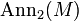# Difference between revisions of "Group cohomology of dihedral group:D8"

View group cohomology of particular groups | View other specific information about dihedral group:D8

## Homology groups for trivial group action

FACTS TO CHECK AGAINST (homology group for trivial group action):
First homology group: first homology group for trivial group action equals tensor product with abelianization
Second homology group: formula for second homology group for trivial group action in terms of Schur multiplier and abelianization|Hopf's formula for Schur multiplier
General: universal coefficients theorem for group homology|homology group for trivial group action commutes with direct product in second coordinate|Kunneth formula for group homology

### Over the integers

The homology groups with coefficients in the ring of integers are as follows:$\! H_p(D_8;\mathbb{Z}) = \left\lbrace \begin{array}{rl} \mathbb{Z}, & \qquad p = 0 \\ \mathbb{Z}/2\mathbb{Z}, & \qquad p \equiv 1 \pmod 4 \\ \mathbb{Z}/8\mathbb{Z}, & \qquad p \equiv 3 \pmod 4 \\ 0, & \qquad p \ne 0, p \ \operatorname{even}\\\end{array}\right.$

As a sequence (Starting$p = 0$), the first few homology groups are:$p$ 0 1 2 3 4 5 6 7 8$H^p(D_8;\mathbb{Z})$$\mathbb{Z}$$\mathbb{Z}/2\mathbb{Z}$ 0$\mathbb{Z}/8\mathbb{Z}$ 0$\mathbb{Z}/2\mathbb{Z}$ 0$\mathbb{Z}/8\mathbb{Z}$ 0

### Over an abelian group

The homology groups with coefficients in an abelian group$M$ are as follows:$H_p(D_8;M) = \left \lbrace \begin{array}{rl} M, & \qquad p = 0 \\ M/2M, & \qquad p \equiv 1 \pmod 4 \\ \operatorname{Ann}_2(M) & \qquad p \equiv 2 \pmod 4 \\ M/8M, & \qquad p \equiv 3 \pmod 4\\ \operatorname{Ann}_8(M), & \qquad p > 0, p \equiv 0 \pmod 4 \\\end{array}\right.$

## Cohomology groups for trivial group action

FACTS TO CHECK AGAINST (cohomology group for trivial group action):
First cohomology group: first cohomology group for trivial group action is naturally isomorphic to group of homomorphisms
Second cohomology group: formula for second cohomology group for trivial group action in terms of Schur multiplier and abelianization
In general: dual universal coefficients theorem for group cohomology relating cohomology with arbitrary coefficientsto homology with coefficients in the integers. |Cohomology group for trivial group action commutes with direct product in second coordinate | Kunneth formula for group cohomology

### Over the integers

The cohomology groups with coefficients in the ring of integers are as follows:$H^p(D_8;\mathbb{Z}) = \left \lbrace \begin{array}{rl} \mathbb{Z}, & \qquad p = 0 \\ \mathbb{Z}/2\mathbb{Z}, & \qquad p \equiv 2 \pmod 4 \\ \mathbb{Z}/8\mathbb{Z}, & \qquad p \ne 0, p \equiv 0 \pmod 4\\ 0, & p \ \operatorname{odd} \\\end{array}\right.$

### Over an abelian group

The cohomology groups with coefficients in an abelian group$M$ are as follows:$H^p(D_8;M) = \left \lbrace \begin{array}{rl} M, & \qquad p = 0 \\ \operatorname{Ann}_2(M), & \qquad p \equiv 1 \pmod 4 \\ M/2M & \qquad p \equiv 2 \pmod 4 \\ \operatorname{Ann}_8(M), & \qquad p \equiv 3 \pmod 4\\ M/8M, & \qquad p > 0, p \equiv 0 \pmod 4 \\\end{array}\right.$

Here$\operatorname{Ann}_2(M)$ denotes the 2-torsion subgroup of$M$ and$\operatorname{Ann}_8(M)$ denotes the 8-torsion subgroup of$M$.